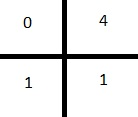Zeta and Thanos
/
No tags
Problem
Editorial
Analytics

Avengers after getting defeated from Thanos called zeta for help.

zeta and Thanos are having a one on one battle in Wakanda. The land of Wakanda consists of a N x M grid.

Every block of the grid can contain elements only from the following set: {0,1,2,3,4,5,6,7,8,9,*}

From a block zeta can move UP, DOWN, LEFT or RIGHT but he cannot go to the block containing * because that block is a warmhole to another dimesion where "Children of Thanos" will kill zeta.

To know his fate before hand, Thanos is using the "Time Stone" to see Q different possibilities.

In each of the Q possibility the position of zeta is (x,y) but that of Thanos changes.

For the ith possibility the position of Thanos is ($x_i, y_i$).

Every time, zeta starts moving towards Thanos and takes the path having shortest distance so that he can reach to Thanos before he snaps his fingers again. :( (RIP Spidey!!)

Distance of the following blocks from (i,j) is 1:

(i+1,j)  (i-1,j)  (i,j+1)  (i,j-1)

If more than one paths are possible with the same shortest distance, zeta takes the path resulting in largest sum of block elements. (If zeta travels from (1,1) -> (1,2) -> (2,2) the sum of block elements will be 5.)Since Thanos is not an easy villian you need to help zeta in finding the shortest distance and its corresponding sum of block elements.

Input Format:

First line contains two space seperated integer N and M.

Next N lines contain M space seperated charcters from the following set: {0,1,2,3,4,5,6,7,8,9,*}

Next line contains to space seperated integers X and Y denoting the positiob of zeta.

Next line contains an integer Q denoting the number of possibilities Thanos sees using the "Time Stone".

Next Q lines contain two space seperated integers ($X_i, Y_i$) denoting Thanos's position in each posibility.

It is guaranteed that initial position of zeta WILL NOT be a '*'. It will always be a block having a number from [0 9].

Output Format:

Print two space seperated integer "Shortest distance to reach thanos" and "corresponding sum of block elements"(Remember that if more than one path with the same shortest distance are possible you need to consider the one with the largest sum of block elements) .

If Thanos is himself standing on * block in any of the posibility, print "-1 -1" (without qoutes).

If zeta is not able to reach Thanos, print "-1 -1" (without qoutes).

INPUT CONSTRAINTS

$1 \le N, M \le 1000$

$1 \le X, X_i \le N$

$1 \le Y, Y_i \le M$

$1 \le Q \le 1000$

SAMPLE INPUT
2 2
7 *
2 5
1 1
2
2 2
2 1
SAMPLE OUTPUT
2 14
1 9
Time Limit: 2.0 sec(s) for each input file.
Memory Limit: 256 MB
Source Limit: 1024 KB

## Contributors

Initializing Code Editor...